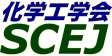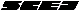## \$B9V1i%W%m%0%i%`!J2q>l!&F|DxJL!K(B

### M\$B2q>l(B \$BBh(B3\$BF|(B

\$B:G=*99?7F|;~!'(B2012-08-20 15:02:17
\$B9V1i(B
\$B;~9o(B
\$B9V1i(B
\$BHV9f(B
\$B9V1iBjL\!?H/I=\$B%-!<%o!<%I(B\$BJ,N`(B
\$BHV9f(B
\$B\$BHV9f(B
\$B%7%s%]%8%&%`(B \$B!c%(%M%k%.!<%7%s%]%8%&%`!d(B
(9:00\$B!A(B10:00)\$B!!(B(\$B:BD9(B \$BARK\(B \$B9@;J(B)
9:00\$B!A(B 9:20M301\$BGQ4~J*G3>F>l\$K\$*\$1\$kEAG.4I\$X\$N3%IUCe5!9=\$N2rL@(B
(\$BL>Bg1!9)(B) \$B!{(B(\$B3X(B)\$B;3K\(B \$B5.J8(B \$B!&(B (\$BL>Bg%(%3%H%T%"(B) \$BI[L\(B \$BM[;R(B \$B!&(B (\$B@5(B)\$B?"LZ(B \$BJ]><(B \$B!&(B (\$BL>Bg1!9)(B) (\$B@5(B)\$B5A2H(B \$BN<(B \$B!&(B (\$BL>Bg%(%3%H%T%"(B) (\$B@5(B)\$B@.@%(B \$B0lO:(B
waste
combustion
ash deposition
S-2300
(\$BCfItBg(B) (\$B3X(B)Jiao Facun \$B!&(B Song Wenjia \$B!&(B (\$B3X(B)Meng Ying \$B!&(B (\$B@5(B)\$B;3ED(B \$BD>?C(B \$B!&(B (\$B@5(B)\$B:4F#(B \$B8|(B \$B!&(B \$B!{(B(\$B@5(B)\$BFs5\(B \$BA1I'(B
Heavy metal vapors
toxicity of deposit
Heterogeneous nucleation
S-2325
9:40\$B!A(B 10:00M303Effect of net-modifier on the microstructure and leaching behavior of slag during high temperature gasification
(\$BCfItBg(B) \$B!{(B(\$B3X(B)\$Br2(B \$B2mU/(B \$B!&(B (\$B@5(B)\$B;3ED(B \$BD>?C(B \$B!&(B (\$B@5(B)\$B:4F#(B \$B8|(B \$B!&(B (\$B@5(B)\$BFs5\(B \$BA1I'(B
slag structure
microstructure
slag stability
S-2438
(10:00\$B!A(B11:00)\$B!!(B(\$B:BD9(B \$BFs5\(B \$BA1I'(B)
10:00\$B!A(B 10:20M304\$B@PC:%,%92=%,%9\$rG3NA\$H\$7\$FMQ\$\$\$?>l9g\$N(BSOFC\$BG3NA6K\$K\$*\$1\$k=tNt2=H?1~\$K4X\$9\$k8&5f(B
(\$B;:Am8&(B) \$B!{(B(\$B@5(B)Kuramoto Koji \$B!&(B (\$B@5(B)Fukushima Toshiyo \$B!&(B (\$B@5(B)Matsuoka Koichi \$B!&(B (\$B@5(B)Suzuki Yoshizo \$B!&(B (\$BL>Bg(B) (\$B@5(B)Yoshiie Ryo \$B!&(B (\$B@5(B)Naruse Ichiro \$B!&(B (\$B4tIlBg(B) (\$B@5(B)Kumabe Kazuhiro \$B!&(B (\$B@5(B)Moritomi Hiroshi
Solid oxide fule cell
coal gasification
trace contaminants
S-2710
10:20\$B!A(B 10:40M305CO2\$B2s<}7?9b8zN((BIGCC\$BMQ4%<0C&N2%W%m%;%9\$K\$*\$1\$kC:AG@O=P1F6A\$HBP:v(B
(\$BEECf8&(B) \$B!{(B(\$B@5(B)\$B>.NS(B \$B@?(B \$B!&(B (\$B@5(B)\$BI[@n(B \$B?.(B \$B!&(B \$BCfHx(B \$B5H?-(B
high temperature sulfur removal
carbon deposition
IGCC with CO2 capture
S-21
10:40\$B!A(B 11:00M306Carbon deposition border in the desulfurization process in IGCC
(\$BJ!2,=wBg(B) \$B!{(BYu Yu \$B!&(B (\$B@5(B)Yuichi Fujioka \$B!&(B (\$BEECf8&(B) (\$B@5(B)Makoto Kobayashi
lCarbon deposition
high temperature sulfur removal
chemical equilibrium
S-2704
(11:00\$B!A(B12:00)\$B!!(B(\$B:BD9(B \$B>.NS(B \$B@?(B)
11:00\$B!A(B 11:20M307\$BE47O5[Ce:^\$rMQ\$\$\$?4%<0C&N2%7%9%F%`\$NC&N2@-G=8!F\$(B
(\$B6eBg9)(B) \$B!{(B(\$B3X(B)\$B5\:j(B \$BM:2p(B \$B!&(B (\$B6eBg1!9)(B) (\$B@5(B)\$B>>7((B \$BMN2p(B \$B!&(B (\$B@5(B)\$BJw85(B \$B2m
IGCC
desulfuration
S-2440
11:20\$B!A(B 11:40M308\$B3h@-%3!<%/%9\$K\$h\$k(BH2S\$B5Z\$S(BCOS\$B5[CeFC@-(B
(\$B4tIlBg9)(B) \$B!{(B(\$B3X(B)\$BJ?0f(B \$B7rM'(B \$B!&(B (\$BL>Bg9)(B) (\$B3X(B)\$BDi(B \$BM:0l(B \$B!&(B (\$B@5(B)\$B>.NS(B \$B?.2p(B \$B!&(B (\$B4tIlBg9)(B) (\$B@5(B)\$BHDC+(B \$B5A5*(B
dry desulfurization
COS conversion
activated cokes
S-2634
11:40\$B!A(B 12:00M309\$B3h@-%3!<%/%9\$r5[Ce:^\$H\$7\$FMQ\$\$\$?4%<0C&N2%W%m%;%9\$K\$*\$1\$k6&B8%,%9\$N1F6A(B
(\$BL>Bg9)(B) \$B!{(B(\$B3X(B)\$BDi(B \$BM:0l(B \$B!&(B (\$B@5(B)\$B>.NS(B \$B?.2p(B \$B!&(B (\$B@5(B)\$BEDn4(B \$BLwGn(B \$B!&(B (\$B@5(B)\$BHDC+(B \$B5A5*(B
gasification
sulfuric compound
S-2397
(13:00\$B!A(B14:00)\$B!!(B(\$B:BD9(B \$B>.NS(B \$B?.2p(B)
13:00\$B!A(B 13:20M313172nmVUV\$B\$rMQ\$\$\$?(BSNCR\$BK!\$N3+H/(B
(\$B4tIlBg1!(B) \$B!{(B(\$B3X(B)\$B6aF#(B \$B8w9@(B \$B!&(B (\$B3X(B)\$BIp;3(B \$B>49((B \$B!&(B (\$B@5(B)\$B?@86(B \$B?.;V(B \$B!&(B (\$B%&%7%*EE5!(B) (\$B6&(B)\$BI)>B(B \$B@k@'(B \$B!&(B (\$B%"%/%H%j!<(B) (\$B6&(B)\$BA}0f(B \$B2j(B \$B!&(B (\$B4tIlBg1!(B) (\$B@5(B)\$B7(It(B \$BOB90(B \$B!&(B (\$B@5(B)\$B
VUV
SNCR
NOx
S-2114
13:20\$B!A(B 13:40M314\$BBg5\$05%W%i%:%^\$rMQ\$\$\$?(BSNCR\$BK!\$N3+H/(B
(\$B4tIlBg1!(B) \$B!{(B(\$B3X(B)\$BD9Fl(B \$B8w(B \$B!&(B (\$B3X(B)\$BIp;3(B \$B>49((B \$B!&(B (\$B@5(B)\$B?@86(B \$B?.;V(B \$B!&(B (\$B_7F#EE5!(B) (\$B6&(B)\$B;01:(B \$BM'5,(B \$B!&(B (\$B%"%/%H%j!<(B) (\$B6&(B)\$BA}0f(B \$B2j(B \$B!&(B (\$B4tIlBg1!(B) (\$B@5(B)\$B7(It(B \$BOB90(B \$B!&(B (\$B@5(B)\$B
Atmospheric plasma
SNCR
NOx
S-2115
13:40\$B!A(B 14:00M315\$B@PL}%3!<%/%9\$N(BKOH\$BIj3h\$GF@\$i\$l\$k3h@-C:\$N:Y9&!&I=LL2=3XFC@-(B
(\$BL>Bg1!9)(B) \$B!{(B(\$B3X(B)\$BCfEg(B \$B424p(B \$B!&(B (\$B@5(B)\$B7&ED(B \$B8w9((B \$B!&(B (\$B@5(B)\$B>>ED(B \$B?N
petroleum coke
activated carbon
S-2429
(14:00\$B!A(B15:00)\$B!!(B(\$B:BD9(B \$BHDC+(B \$B5A5*(B)
14:00\$B!A(B 14:20M316\$BMO:^Cj=P%U%i%/%7%g%M!<%7%g%sK!\$K\$h\$k@PC:!&G47k:`\$N%3!<%/%92=5sF0M=B,\$N;n\$_(B
(\$B5~Bg9)(B) \$B!{(B(\$B@5(B)\$BiCED(B \$BN40l(B \$B!&(B (\$B3X(B)\$B9bEg(B \$B7r?M(B \$B!&(B (\$B@5(B)\$B;01:(B \$B9'0l(B \$B!&(B (\$B?@8M@=9](B) \$B1|;3(B \$B7{9,(B \$B!&(B \$B_@8}(B \$BbC4p(B \$B!&(B \$B<58M(B \$B5.MN(B
Solvent extraction
Coking coal
Binder
S-2646
14:20\$B!A(B 14:40M317\$B2:OB\$JMO:^=hM}\$K\$h\$j2~
(\$B5~Bg9)(B) \$B!{(B(\$B3X(B)\$B1|ED(B \$B8-;KO:(B \$B!&(B (\$B@5(B)Li Xian \$B!&(B (\$B@5(B)\$BiCED(B \$BN40l(B \$B!&(B (\$B@5(B)\$B;01:(B \$B9'0l(B
carbon fiber
stabilization
\$B"#(Bextraction
S-2849
14:40\$B!A(B 15:00M318Liquefaction of extracts obtained from low-rank coal and biomass extraction at mild condition
(Kyoto U.) \$B!{(B(\$B@5(B)Li Xian \$B!&(B (\$B3X(B)Priyanto Dedy Eka \$B!&(B (\$B@5(B)Ashida Ryuichi \$B!&(B (\$B@5(B)Miura Kouichi
low-rank coal/biomass
liquid fuel
S-2977
(15:00\$B!A(B16:20)\$B!!(B(\$B:BD9(B \$BiCED(B \$BN40l(B)
15:00\$B!A(B 15:20M319\$B%P%\$%*%^%9!&@PC::.9g%,%92=\$K\$h\$k1U2=G3NA9g@.MQ%,%9@=B\$\$K4X\$9\$k8&5f(B
(\$B4tIlBg9)(B) \$B!{(B(\$B3X(B)\$B9)F#(B \$BAo0lO:(B \$B!&(B (\$B@5(B)\$BHDC+(B \$B5A5*(B
gasification
coal
biomass
S-2395
15:20\$B!A(B 15:40M320\$B%;%k%m!<%9\$N9ZAGE|2=\$K\$*\$1\$k(BCBH1\$B\$H(BCBH2\$B\$NAj>h8z2L(B
(\$BF|4x(B) \$B!{(B(\$B@5(B)\$B \$B!&(B \$BCSXf(B \$B?? \$B!&(B \$B2-Ln(B \$B>MJ?(B
biomass
enzyme
hydrolysis
S-263
15:40\$B!A(B 16:00M321\$B%P%\$%*%^%9\$N9ZAGE|2=\$K\$*\$1\$k9ZAG\$NHs@8;:E*5[Ce8=>](B
(\$BF|4x(B) \$B!{CSXf(B \$B?? \$B!&(B (\$B@5(B)\$B \$B!&(B \$B2-Ln(B \$B>MJ?(B
biomass
enzyme
hydrolysis
S-264
16:00\$B!A(B 16:20M322\$BKlJ,N%MOG^Cj=P\$K\$h\$kGQ=a3jL}\$N:F@8
(\$BL>Bg1!9)(B) \$B!{(B(\$B3X(B)\$BJR2,(B \$BD>Li(B \$B!&(B (\$BL>Bg%(%3%H%T%"(B) (\$B@5(B)\$B@.@%(B \$B0lO:(B \$B!&(B (\$BL>Bg1!9)(B) (\$B@5(B)\$B5A2H(B \$BN<(B \$B!&(B (\$BL>Bg%(%3%H%T%"(B) (\$B@5(B)\$B?"LZ(B \$BJ]><(B \$B!&(B \$BI[L\(B \$BM[;R(B
refinement
waste lubricant
membrane
S-2371

\$B9V1i%W%m%0%i%`(B
\$B2=3X9)3X2q(B \$BBh(B44\$B2s=)5(Bg2q(B(C) 2012 \$B8x1W
Most recent update: 2012-08-20 15:02:17
For more information contact \$B2=3X9)3X2qElKL;YIt(B \$BBh(B44\$B2s=)5(Bg2q(B \$BLd\$\$9g\$;78(B
E-mail: inquiry-44fwww3.scej.org
This page was generated byeasp 2.28; proghtml 2.28c (C)1999-2011 kawase